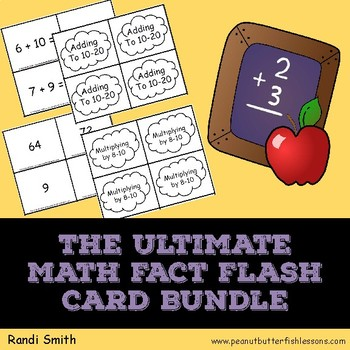DID YOU KNOW:
Seamlessly assign resources as digital activities

Learn how in 5 minutes with a tutorial resource. Try it Now

Learn More# The Ultimate Math Fact Flash Card BundleK - 4th, Homeschool
Subjects
Standards
Resource Type
Formats Included
• PDF
Pages
144 pages

### Description

Do your children need some extra help in memorizing AND recalling their addition, subtraction, multiplication and division facts? These flash cards with matching answer cards can be used to play a variety of games such as memory, go fish, slap a fact and more.

They are organized based upon number sense principles such as teaching doubles, plus and minus 1 and 2 and deriving multiplication and division facts from previously learned multiplication facts.

These fact cards are perfect for math review for the summer months when learning should be kept fun!

Total Pages
144 pages
N/A
Teaching Duration
N/A
Report this Resource to TpT
Reported resources will be reviewed by our team. Report this resource to let us know if this resource violates TpT’s content guidelines.

### Standards

to see state-specific standards (only available in the US).
Fluently multiply and divide within 100, using strategies such as the relationship between multiplication and division (e.g., knowing that 8 × 5 = 40, one knows 40 ÷ 5 = 8) or properties of operations. By the end of Grade 3, know from memory all products of two one-digit numbers.
Fluently add and subtract within 20 using mental strategies. By end of Grade 2, know from memory all sums of two one-digit numbers.
Add and subtract within 20, demonstrating fluency for addition and subtraction within 10. Use strategies such as counting on; making ten (e.g., 8 + 6 = 8 + 2 + 4 = 10 + 4 = 14); decomposing a number leading to a ten (e.g., 13 - 4 = 13 - 3 - 1 = 10 - 1 = 9); using the relationship between addition and subtraction (e.g., knowing that 8 + 4 = 12, one knows 12 - 8 = 4); and creating equivalent but easier or known sums (e.g., adding 6 + 7 by creating the known equivalent 6 + 6 + 1 = 12 + 1 = 13).
Fluently add and subtract within 5.